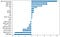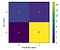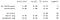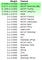# Detecting Parkinson's Disease with Voice Analysis

Parkinson’s Disease (PD) develops slowly over the span of years, and there are currently no lab tests or biomarkers to detect it. Instead, a diagnosis relies on an overall evaluation of physical symptoms, such as tremors, gait, and slowness of movement. It can take days, or years to diagnose PD. However, there are promising studies in using voice analysis to accurately diagnose patients.

A study by Max A. Little, Patrick E. McSharry, Eric J. Hunter, Lorraine O. Ramig (‘Suitability of dysphonia measurements for telemonitoring of Parkinson’s disease’, 2008), examined vocal impairment as a symptom of PD. The impairment may be reduced volume, breathiness, or vocal tremor, among others. The study took voice recordings from thirty one subjects, twenty three which had PD, and measured their variations in frequency and amplitude. If a computer could diagnose PD accurately based on a voice sample, it could open the door to widespread or early detection, possibly through smartphone apps.

Using their dataset, I built a machine learning model to predict if the subject had PD, a binary classification model. The target was removed from the training data to avoid leakage. After creating each model, I reviewed its feature importances to confirm none of the remaining features were leaks. The majority baseline to beat was 75%, which was the number of subjects who had PD.

Since this was a small dataset (195 observations), I held out 10% of the observations to serve as my test set, and used Cross Validation to train and validate the other 90%. I stratified the split to maintain the same proportion of PD subjects in both the train and test set, which is appropriate for a small and imbalanced dataset.

Here are the different models I tested:

# Logistic Regression

Logistic Regression is a simple binary classification model. I standardized the data, fit the model, and evaluated the accuracy score using k-folds cross validation. The mean score of ten k-folds was 85%. I used a randomized cross validation search to find a better set of hyperparameters, which brought the accuracy score up to 87%. As a reminder, the baseline was 75%.

Below is a visualization of the model’s coefficients. There were twenty two features observed in the study, various vocal characteristics’ frequencies and amplitudes. The larger positive and negative coefficients indicate the features are significant to the model. The features with coefficients that are closer to zero are less significant.Coefficients for the Logistic Regression model

# Random Forest Classifier

Next was a Random Forest Classifier model, where I also used a cross validation randomized search to tune the hyperparameters. The resulting model gave a score of 91%, besting the Logistic Regression model (87%).

Because the dataset is imbalanced, I plot a confusion matrix to check the F1 score on the test data. This was to make sure that the model’s strong accuracy scores weren’t misleading.Confusion matrix

The test data had a weighted average F1 score of 89%. The weighted average is a better metric when working with imbalanced data, compared to the macro average of 84%. The high F1 score gave me confidence in the model’s high accuracy scores.F1 Score

# XGBoost

Lastly, I created an XGBoost model and used early stopping to optimize the hyperparameters. This model’s accuracy score was 93%, better than the Random Forest Classifier model of 91%.

Having selected the best performing model, I retooled the model once more to streamline it for future use. Using Permutation Importance, I identified the features that contributed to the model, and eliminated the rest from the training data.Permutation Importance

The second XGBoost model used only the five highlighted features and still produced the same accuracy score.

# The Test Data Speaks

The most important test that the models must pass is the test on unknown data, so I employed the holdout test data on the final XGBoost model. The accuracy score was 90%.

Here is a plot of the ROC (Receiver Operating Characteristic), showing how often the XGBoost model made correctly predicted probabilities. The metric used to measure this plot is the AUC (Area Under the Curve), which was 96%, (out of a possible 100% accuracy).

Below is a Shapley Plot demonstrating the effect each feature has in determining the predicted probability of the target. Closer to one is a higher probability of having PD.

# Conclusion

As these models demonstrate, you can use voice analysis to diagnose PD with a high accuracy. After experimenting with different models, the final model had the benefit of being more accurate, and computationally inexpensive by only requiring 5 features.

Data Science student at Lambda School

## More from Temsy Chen

Data Science student at Lambda School